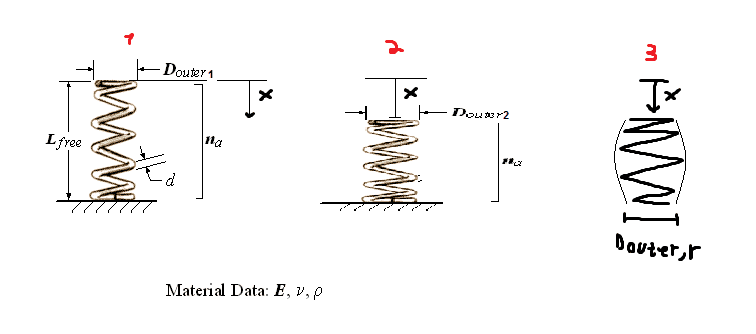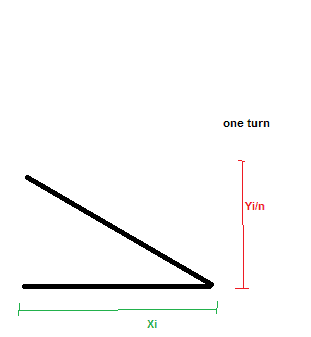# Spring diameter change under pressure

dislect
Hi guys,

I have a question and I would love some guidance or external reference for relevant information.

I have a normal compression spring (see attached pic - 1), I apply a force to compress it by x length.
I want to create a formula connecting between the small increase of the outer diameter of the spring and the length x.1. Would the shape of the spring look like in pic - 2 by a good approximation or more similar to pic - 3, meaning the outer diameter is not the same throughout the length of the spring when compressed and is of a parabolic shape?
2. Help figuring out how to connect between x and the new Douter?

Thanks a lot guys,

Have a good day

Mentor
If the end diameters were not constrained laterally, it would be like in Figure 2. Look up the equation for a helix. The constraint is that the developed length of wire in the helix is unchanged when you compress.

Chet

dislect
Hi Chestermiller,
I'm sorry but I don't follow. What do you mean equation for a helix?
What is "the developed length of wire" - The total length of the wire if I was to straighten it?

I came across this info of the diameter change (http://springipedia.com/compression-general-design.asp) with the formula:
O.D.expension= sqr root (D^2+ d + (p^2-d^2/(Pi^2))-OD
DESIGN HANDBOOK Engineering Guide To Spring Design, 1987 Edition, Page 31, Associated Spring - Barnes Group Inc

But I really want to know how it was developed, plus it has nothing to do with the compression length I called x

Last edited:
Mentor
Hi Chestermiller,
I'm sorry but I don't follow. What do you mean equation for a helix?
What is "the developed length of wire" - The total length of the wire if I was to straighten it?

I came across this info of the diameter change (http://springipedia.com/compression-general-design.asp) with the formula:
O.D.expension= sqr root (D^2+ d + (p^2-d^2/(Pi^2))-OD
DESIGN HANDBOOK Engineering Guide To Spring Design, 1987 Edition, Page 31, Associated Spring - Barnes Group Inc

But I really want to know how it was developed, plus it has nothing to do with the compression length I called x
I don't really know what the parameters in the above equation are. If I were writing the equation for a helix, I would write something like the parametric equations

r = Helix radius = const.
θ = θ(s)
z = z(s)

where s is the developed length along the helix. If $\phi$ is the helix angle (which is constant), then

$$\theta=\frac{s}{r}\cos \phi$$
$$z=s\sin \phi$$

If you stretch the spring, z gets longer, while the total developed length s and the total number of turns (as characterized by the the total spiral angle θ) is held constant. So how does r change as a function of z, holding θ and s constant?

Your are correct that the developed length s is the total length of the wire if you straightened it.

dislect
I would say that just playing with equations gives me r=$\frac{z}{θ*tan∅}$

But I'm not sure I understand what θ and ∅ stands for, can you refer me to a sketch showing the dimensions? I want to translate these to typical spring dimensions such as number of coils, pitch and so on.

2021 Award
Consider a spring of n turns, axial length y and wire mid-diameter x = (ID + OD) / 2.
Initially axial length is yi and mid-diameter = xi
The wire length per turn is Sqrt( ( Pi * xi )^2 + (yi / n)^2 )
The spring length y will control the mid-diameter x.

Sqrt( ( Pi * x )^2 + (y / n)^2 ) = Sqrt( ( Pi * xi )^2 + (yi / n)^2 )
( Pi * x )^2 = ( Pi * xi )^2 + (yi / n)^2 – (y / n)^2
Pi * x = Sqrt( ( Pi * xi )^2 + (yi / n)^2 – (y / n)^2 )

Therefore x = Sqrt( ( Pi * xi )^2 + (yi / n)^2 – (y / n)^2 ) / Pi

dislect
Hi Baluncore, thank you for the rapid reply.
Again, i'm struggling understanding the dimensions (axial length yi? xi?)
Could you please take a random spring jpeg and mark the dimensions?

thanks a lot and sorry for the trouble

Mentor
I would say that just playing with equations gives me r=$\frac{z}{θ*tan∅}$

But I'm not sure I understand what θ and ∅ stands for, can you refer me to a sketch showing the dimensions? I want to translate these to typical spring dimensions such as number of coils, pitch and so on.

θ is 2π times the number of turns.
tan(phi) is the pitch divided by 2πr

In the analysis I did, I was just following the centerline of the coil, which, for a wire diameter that is small compared to the spring diameter, is adequate. If you take the equations that I gave you and integrate, you get simply

$$z^2+(r\theta)^2=z_0^2+(r_0\theta)^2=s^2$$

where z is the total length of the spring, and r is the radius of the spring. So, if we differentiate, we obtain:

z0dz+θ2r0dr=0
The superficial strains in the axial and radial directions, respectively are
εz=dz/z0
εr=dr/r0

So, $$z_0^2\epsilon_z+\theta^2r_0^2\epsilon_r=0$$

So, using the relationships I presented in a previous post, we obtain:

$$\epsilon_r=-\tan^2\phi\epsilon_z$$
where $\phi$ is the helix angle of the spring.

Last edited:
2021 Award
dislect said:
Hi Baluncore, thank you for the rapid reply.
Again, i'm struggling understanding the dimensions (axial length yi? xi?)
Could you please take a random spring jpeg and mark the dimensions?
See attached.
Also;
OD = outside diameter of coil spring
ID = inside diameter of coil spring
For a wire diameter d, we then get
OD = x + d and
ID = x – d.

#### Attachments

Mentor
I made some changes in my post #8 to correct an error, and to complete the analysis. It shows that the superficial strain in the radial direction is equal to minus the superficial strain in the axial direction time the tangent square of the initial helix angle.

Chet

dislect
Hi Baluncore, Thank you for the pic!
So you say that the wire length on a single turn is equal when the spring is compressed and when it is free, right?
How did you come by the equation Sqrt( ( Pi * xi )^2 + (yi / n)^2 ) ?

Chestermiller, It was kind of hard for me to follow once you got to differentiating. I understood what you've done up to that point.

Mentor
Chestermiller, It was kind of hard for me to follow once you got to differentiating. I understood what you've done up to that point.

Check out the equation I wrote for s^2 in post #8. That tells it all.

•1 person
2021 Award
dislect said:
How did you come by the equation
The flattened surface of the cylinder that contains one turn of the spring is a rectangle.
It has a width that is the circumference of the cylinder, = Pi * x.
It has a length of one turn which is the spring pitch, = yi / n.
The wire runs diagonally across that surface.
Pythagoras said the length of the diagonal was the root of the sum of the squares.
Hence length of one turn of the relaxed spring is; = Sqrt( ( Pi * xi )^2 + (yi / n)^2 )

•1 person
dislect
Baluncore I can't quite imagine it from the view you're talking about.
I understand the length but not the width.
Are you seeing this as something like this?
If so i dont understand why pi*xi and not xi•